Robinson-Schensted correspondence

(diff) ← Older revision | Latest revision (diff) | Newer revision → (diff)

A bijection between certain sequences of numbers and pairs of Young tableaux (cf. Young tableau), defined by means of a combinatorial algorithm. In its basic form, the Robinson–Schensted correspondence gives a bijection between the symmetric group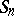, whose elementsare represented by the sequences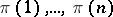, and pairs of standard Young tableaux of orderwith equal shapes. The two tableaux are usually referred to asand, or as the-symbol and-symbol of the permutation.

The-symbol can be obtained by repeatedly applying the following so-called Schensted insertion procedure to the terms of the sequence, from left to right, to enter them into an initially empty tableau. To insert a number, it is placed in the first row of, either by replacing the first entry greater thanin that row, or if there is no such entry, by adding it to the end of the row. If a number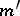was replaced,is placed in the next row using the same rule, and so on, until at some step no number is replaced. The-symbol ofrecords the successive shapes ofby having entryin the square added to the shape ofby insertion of; the final value ofis the-symbol of. E.g., forone has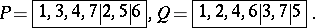Given standard Young tableaux,of the same shape, this algorithm can be reversed to determine the permutationwith these- and-symbols.

The correspondence has a fundamental but non-trivial symmetry: replacingbyinterchangesand. There are more symmetries, which require another algorithmically defined correspondence, namely a shape-preserving involution of the set of standard Young tableaux that is due to M.-P. Schützenberger [a9]. For instance, reversing the sequence corresponding totransposes bothand, while also applying this involution to. Details can be found in [a3] and [a6].

A generalization of this correspondence is obtained by allowing arbitrary sequences ofnumbers taken from some fixed set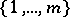; the same algorithm then defines a bijection between the set of such sequences and pairs,of equal shape, whereis still a standard Young tableau of order, butis now a semi-standard Young tableau (see Young tableau) with entries in. This form gives the decomposition of the character of the representation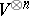of the general linear group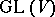with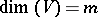, into irreducible characters (which are Schur polynomials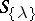, see Symmetric polynomial): each standard Young tableauof shapecorresponds to a factor, and each semi-standard Young tableauof shapeand type (or weight)corresponds to a term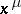of. A further generalization, which restores the symmetry betweenand, is defined in [a2], and an even further generalization, in which,, andare all pictures, is defined in [a12]; it probably provides the most natural setting for the Robinson–Schensted correspondence.

Having the same-symbol defines an equivalence relation on sequences of numbers called plactic equivalence. It is generated by relations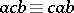for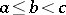andfor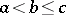(cf. [a2]); therefore, the set of equivalence classes forms a monoid under concatenation, called the plactic monoid, which has many interesting properties, see [a4].

There exist other algorithmic descriptions of the correspondence than the one given above. In fact, the description originally given by A. Robinson in an (incomplete) attempt to prove the Littlewood–Richardson rule [a7] is rather different. Also, a very simple game, called the jeu de taquin, defined by Schützenberger [a10] gives a non-deterministic procedure for computing the-symbol. Besides providing useful enumerative identities, the correspondence itself has various useful interpretations, e.g., it defines the Kazhdan–Lusztig cells in the symmetric groups [a1], and it has interpretations in terms of the geometry of the flag manifold of general linear groups [a11], and of straightening [a5].